V4.1.1 July 19, 2021

Added: New Goblin Invasion Event Feat.

Balance Changes

DM2 (Devil Tyrant)

Effect: The three highest building tiers count more based on time spent as Evil in this Reincarnation.

Old Formula: (0.6 * x ^ 0.6)%, where x is time spent as Evil this Reincarnation.

New Formula: (0.4 * x ^ 0.6)%, where x is time spent as Evil this Reincarnation.

Note: Reverted formula back to where it was in v4.0

TT4 (Titan Drill)

Effect: Increase the base production of Warrior Barracks / Slave Pens / Deep Mines by +9635, and Cathedrals / Dark Temples / Monasteries by +98000 (based on your Alignment).

Added Effect: Also increases building production of these tiers by 1000%.

TT8 (Heavy Coins)

Old Effect: Increases Royal Exchanges production bonus by an additional 40% each, and reduces Royal Exchange cost multiplier by 0.01.

New Effect: Increases Royal Exchanges production bonus by an additional 100% each, and reduces Royal Exchange cost multiplier by 0.01.

Old Effect: Increase clicking reward by 1000% per active spell.

Old Formula: ((x * 1000) ^ (A + 1))%, where x is the amount of active spells you have and A your Ascension.

New Effect: Increase clicking reward by 100% per active spell.

New Formula: ((x * 100) ^ (A + 1))%, where x is the amount of active spells you have and A your Ascension.

C3100 (Engineering)

Old Requirement: 15 days as Mercenary (across all Reincarnations).

New Requirement: 12 days as Mercenary (across all Reincarnations).

Makers Perk 2

Old Challenge: Have at least 350,000 buildings within 45 seconds of a new run.

New Challenge: Have at least 300,000 buildings within 45 seconds of a new run.

V4.1.0 June 28, 2021

Effect: Once you purchased 2 different Vanilla Legacies, you will be able to select their specific Legacy Combo upgrade.

Coin Cost: 1 Tvg (1e72).

Faction Coin Cost: 1 Dc (1e33) of both Factions.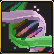Fairy/Elf - Mystic Forest

Effect: Increase the production of all buildings based on the amount of assistants you own.

Formula: (2 * ln(1 + x) ^ 2)%, where x is the amount of assistants you own.Fairy/Angel - Dream Realm

Effect: Increase Mana Regeneration based on time spent as Good in this Reincarnation.

Formula: (0.7 * x ^ 0.7)%, where x is time spent as Good in this Reincarnation.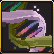Fairy/Goblin - Artifact Caveau

Effect: Increase the production of all buildings based on artifacts found.

Formula: (x ^ 0.95)%, where x is the number of artifacts found.Fairy/Undead - Twisting Nether

Effect: Gain a new spell with the same name (Duration: 20 seconds, Mana Cost: 1 M (1e6), can be cast up to Tier 99). While active, increases Lineage Level by +1 per Tier.

Note: The Lineage Level increase is only a 'count more' effect and does not affect base lineage in any way.

Note: Twisting Nether counts as a faction spell, but has no alignments for upgrades such as Djinn Challenge 5.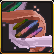Fairy/Demon - Brooding Abyss

Effect: Chaotically increase assistants based on amount of alignments among the active spells you have.

Formula: ((a ^ 1.5 + b ^ 1.5 + c ^ 1.5 + d ^ 1.5 + e ^ 1.5 + f ^ 1.5)^((1 + sum(a, b, c, d, e, f)) * 0.5))%, where a - f represent the six alignments.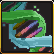Elf/Angel - Sacred Glade

Effect: Increase all spells duration based on clicks made in this game.

Formula: (2.4 * x ^ 0.4)%, where x is the amount of clicks made in this game.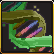Elf/Goblin - Greed Factory

Effect: Multiplicatively increase Faction Coin find chance based on Tax Collections casts in this game.

Formula: (2.5 * ln(1 + x) ^ 2.5)%, where x is the amount of Tax Collection casts in this game.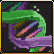Elf/Undead - Swamp of Sorrows

Effect: Activity time of all spells counts more based on Maximum Mana.

Formula: (2 * ln(1 + x) ^ 2.25)%, where x is your Maximum Mana.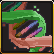Elf/Demon - Forbidden Grove

Effect: Autoclicks per second based on the amount of Unique Buildings you own.

Effect: Also generates the same amount of clicks while offline.

Formula: +(1 + 1.5 * x ^ 0.2), where x is the amount of Unique Buildings you own.

Effect: Also increases production of Unique Buildings based on Autoclicks this game.

Formula: (2 * log10(1 + x) ^ 2)%, where x is Autoclicks made this game.

Note: 'Clicks count more' effects are not affecting this upgrade.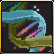Angel/Goblin - Golden Heaven

Effect: Increase the production of all buildings based on Gold owned.

Formula: (log10(1 + x) ^ 2)%, where x is the current amount of Gold you own.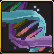Angel/Undead - Dark Sanctum

Effect: Multiplicatively increase Maximum Mana based on time spent as Order in this Reincarnation.

Formula: (0.7 * x ^ 0.7)%, where x is time spent as Order in this Reincarnation.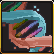Angel/Demon - Eternal Battlefield

Effect: Increase the production of Evil Buildings based on time spent as Good in this Reincarnation, and vice-versa. Does not affect Neutral Buildings

Formula: (0.7 * x ^ 0.7)%, where x is time spent as Good/Evil in this Reincarnation.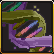Goblin/Undead - Homunculus Lab

Effect: Increase Research Budget based on the least time spent between Order and Balance in this Reincarnation.

Formula: +(2000 + 0.7 * ln(min(x , y)) ^ 3.5)%, where x and y is time spent as Order and Balance respectively in this Reincarnation.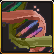Goblin/Demon - Corruption Zone

Effect: Increase the production of Non-Unique buildings based on the duration of your longest spell.

Formula: (0.8 * x ^ 0.8)%, where x is the duration of your longest spell.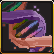Undead/Demon - Frozen Wastes

Effect: Increase Offline Production based on time spent offline in this Reincarnation.

Formula: (0.7 * x ^ 0.7)%, where x is time spent offline in this Reincarnation.

New Trophy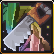Novice Combinator

Requirement: R220+, Purchase Legacies from 4 different factions in a single game.

Cost: 1 Qavg (1e75)

Effect: Increase production for Good or Evil factions based on time spent with the least used alignment.

Formula: (50 + 0.7 * x ^ 0.7)%, where x is time spent with your least used alignment.

New Researches

Note: Research Points now capped at 13,000.

Spellcraft

S11875 (Arcane Efficiency)

Effect: While both Holy Light and Fairy Chanting are active, reduce the cost of all spells by 85%.

Note: Catalyst can be used as one of the spells.

S12250 (Necrodoomancy)

Effect: Reincarnations count more based on time spent as Undead in this Reincarnation.

Formula: (0.5 * x ^ 0.5)%, where x is time spent as Undead in this Reincarnation.

Craftsmanship

C11875 (Plating)

Effect: Unique Buildings count more for Call to Arms purposes based on Moon Blessing activity time in this Reincarnation.

Formula: +(2 * x ^ 0.8), where x is Moon Blessing activity time in this Reincarnation.

Note: This is an additive bonus and is thus not affected by any multiplicatives such as Good Warcry.

C12250 (Compression)

Effect: Brainwave increases Faction Coin find chance progressively over its duration.

Formula: +(floor(1.5 * ((x + y) / 60) ^ 2.25)%, where x is Brainwave runtime and y is Headstart in seconds.

Divine

D11875 (Virtues)

Effect: Increase Tax Collection worth based on the highest amount of Mountain Palaces you built in this Reincarnation.

Formula: (2.5 * x ^ 0.5)%, where x is the highest amount of Mountain Palaces built in this Reincarnation.

D12250 (Enochian)

Effect: Multiply Angel Coins found based on God's Hand activity time in this Reincarnation.

Formula: *(10 + 0.7 * x ^ 0.7)%, where x is God's Hand activity time in this Reincarnation.

Economics

E11875 (Overworking)

Effect: Goblin's Greed casts additional Tax Collection per second based on the amount of assistants you have.

Formula: +(0.85 * x ^ 0.85), where x is the amount of assistants you have.

Note: Catalyst can also trigger this effect.

E12250 (Oilery)

Effect: While both Lightning Strike and Gem Grinder are active, multiplicatively increase the production bonus from Gems based on the duration of your longest spell.

Formula: (0.8 * x ^ 0.8)%, where x is the duration of your longest spell.

Alchemy

A11875 (Liquefaction)

Effect: Increase the production of the three highest building tiers based on Hellfire Blast activity time in this Reincarnation.

Formula: (0.7 * x ^ 0.7)%, where x is Hellfire Blast activity time in this Reincarnation.

A12250 (Folgoration)

Effect: While both Diamond Pickaxe and God's Hand are active, multiplicatively increase Mana Regeneration and Faction Coin find chance based on the amount of Excavations made in this Reincarnation, including resets.

Formula: (15 + 1.75 * (x * y) ^ 0.75)%, where x is the amount of Excavations and y the amount of Excavation resets made in this Reincarnation respectively.

Warfare

W11875 (Scouting)

Effect: Buildings count more based on the maximum amount of Grand Balance targets you had in this Reincarnation.

Formula: (1.25 * x ^ 1.25)%, where x is the maximum amount of Grand Balance targets you had in this Reincarnation.

Note: Amount of targets can be seen at Druid Challenge Reward, but needs an active Grand Balance to update.

W12250 (Raiding)

Effect: Increase the production of all buildings based on the highest Combo Strike counter you had in this Reincarnation.

Formula: (x ^ 0.7)%, where x is the highest Combo Strike counter in this Reincarnation.

Forbidden

F12000 (Revelation)

F12500 (Avatars)

Effect: Multiplactively increase assistants based on the amount of different Factions in your Legacies.

Formula: (50 * x ^ 2.5)%, where x is the amount of different Factions in your Legacies.

Balance Changes

Reincarnation Power

New R230 Power

Effect: While playing as your least used alignment in this Reincarnation, increase the production of all buildings based on Reincarnations made.

Formula: (10 * R)%

Fairy

FR2 (Fairy Workers)

Old Effect: Increase the production of Farms, Inns and Blacksmiths by 15,000%.

New Effect: Increase the production of Farms, Inns and Blacksmiths by 10,000%.

FR6 (Spellsmith)

Effect: Blacksmiths also increase your Mana Regeneration rate.

Old Formula: +(x ^ 0.25), where x is the amount of Blacksmiths you own.

New Formula: +(2.75 * x ^ 0.275), where x is the amount of Blacksmiths you own.

FR9 (Swarm of Fairies)

Effect: You gain additional assistants based on the amount of Farms, Inns and Blacksmiths you own.

Old Formula: +(0.7 * x ^ 0.5), where x is the amount of Farms, Inns and Blacksmiths you own.

New Formula: +(1.2 * x ^ 0.6), where x is the amount of Farms, Inns and Blacksmiths you own.

Elven

EL1 (Elven Mint)

Old Effect: The base chance to find Faction Coins is doubled.

New Effect: The base chance to find Faction Coins is increased by a multiplicative 150%.

EL2 (Elven Treasure Casing)

Effect: Increase base clicking reward by +25,000 per trophy, and increase chance to find faction coins based on the amount of trophies you unlocked.

Old Formula: +floor((1+0.05 * x) ^ 0.95)%, where x is the amount of Trophies unlocked.

New Formula: +((1 + 0.1 * x) ^ 0.95)%, where x is the amount of Trophies unlocked.

EL3 (Ancient Clicking Arts)

Effect: Increase Mana Regeneration based on the amount of clicks made in this Reincarnation.

Old Formula: +(2 * log10(1 + x)), where x is the amount of clicks made in this Reincarnation.

New Formula: +(2 * log10(1 + x) ^ 1.25), where x is the amount of clicks made in this Reincarnation.

EL5 (Elven Efficiency)

Effect: Increase Royal Exchange bonus based on Faction Coins found in this game.

Old Formula: +(2 * log10(1 + x) ^ 2)%, where x is Faction Coins found this game.

New Formula: +(2 * ln(1 + x) ^ 2)%, where x is Faction Coins found this game.

EL8 (Elven Luck)

Effect: Each time you click for money you have 1% chance to gain coins equal to 250000% of your production, also generating Faction Coins based on reincarnation made.

Formula (Coin reward): (2500 * (x + y)), where x is the production of all buildings, and y is the production of one assistant.

New Effect: Assistants now have the same chance to trigger Elven Luck once every second. Elven Lucks triggered this way have their coin reward multiplied by the amount of assistants you have.

EL10 (Wooden Dice)

Effect: Increase Elven Luck chance to activate and its effects based on the highest amount of Arboreal Cities you own in this Reincarnation.

Old Elven Luck Formula: +(x ^ 0.3)%, where x is highest amount of Arboreal Cities this Reincarnation.

New Elven Luck Formula: +(x ^ 0.4)%, where x is highest amount of Arboreal Cities this Reincarnation.

Old Production Formula: (32 * x ^ 0.8)%, where x is highest amount of Arboreal Cities this Reincarnation.

New Production Formula: (40 * x ^ 0.8)%, where x is highest amount of Arboreal Cities this Reincarnation.

Old Faction Coin Formula: (3 * x ^ 0.75)%, where x is highest amount of Arboreal Cities this Reincarnation.

New Faction Coin Formula: (7.5 * x ^ 0.75)%, where x is highest amount of Arboreal Cities this Reincarnation.

Angel

AN2 (Angelic Determination)

Effect: Increase the production of all buildings based on spells cast in this game.

Old Formula: (6 * ln(1 + x) ^ 2)%, where x is spells cast this Game.

New Formula: (4 * ln(1 + x) ^ 2)%, where x is spells cast this Game.

AN6 (Archangel Feathers)

Old Effect: Multiplicatively increase Mana Regeneration by 100%.

New Effect: Multiplicatively increase Mana Regeneration by 50%.

AN7 (Magical Gates)

Effect: Increase Maximum Mana based on time spent as Good in this Reincarnation.

Old Formula: +(7 * x ^ 0.7), where x is time spent as Good this Reincarnation.

New Formula: +(3.5 * x ^ 0.7), where x is time spent as Good this Reincarnation.

AN9 (Wings of Liberty)

Effect: Increase the production of all buildings based on Mana Regeneration.

Old Formula: (6 * ln(1 + x) ^ 2)%, where x is your Mana Regeneration.

New Formula: (4 * ln(1 + x) ^ 2)%, where x is your Mana Regeneration.

Goblin

Effect: Increase the production of all buildings based on the amount of Evil buildings you own.

Old Formula: (2 * x ^ 0.6)%, where x is the amount of Evil buildings you own.

New Formula: (2 * x ^ 0.7)%, where x is the amount of Evil buildings you own.

GB4 (Black Market)

Old Effect: Production bonus from gems is doubled.

New Effect: Production bonus from Gems is increased by a multiplicative 150%.

GB5 (Goblin Economists)

Old Effect: Mutiplicatively increase Mana Regeneration based on upgrades purchased.

Old Formula: (x ^ 0.9)%, where x is upgrades purchased.

New Effect: Multiplicatively increase Mana Regeneration based on Gold owned.

New Formula: (0.75 * log10(1 + x) ^ 1.25)%, where x is Gold owned.

Old Effect: Gems and upgrades count 200% more.

New Effect: Increase the production of all buildings based on the worth in seconds of your Tax Collection spell.

Formula: (12 * ln(1 + x) ^ 3)%, where x is the seconds of your Tax Collection.

GB9 (Green Fingers Discount)

Effect: Gain coins randomly every 10 minutes. Also works while being offline.

Old Formula: (randRange(1 , 1200) * x), where x is your total (buildings + assistants) coin production per second, does not include spells.

New Formula: (randRange(1 , 2500) * x), where x is your total (buildings + assistants) coin production per second, including spells.

UD4 (Unholy Rituals)

Effect: Increase offline production based on time spent being offline in this Reincarnation.

Old Formula: (0.3 * x ^ 0.7)%, where x is your offline time this Reincarnation.

New Formula: (0.4 * x ^ 0.8)%, where x is your offline time this Reincarnation.

UD5 (Corpse Supply)

Old Effect: Gain additional assistants based on the highest amount of Necropolises you built this Reincarnation.

Old Formula: +(2.5 * x ^ 0.5), where x is the highest amount of Necropolises you built this Reincarnation.

New Effect: Gain additional assistants based on time spent being offline in this Reincarnation.

New Formula: +(0.4 * x ^ 0.6), where x is the time spent offline in this Reincarnation.

UD6 (Plagued Buildings)

Effect: Increase the production of all buildings based on time spent in this game.

Old Formula: (0.6 * x ^ 0.7)%, where x is time spent this game.

New Formula: (0.75 * x ^ 0.75)%, where x is time spent this game.

Demon

DM2 (Devil Tyrant)

Effect: The three highest building tiers count more based on time spent as Evil in this Reincarnation.

Old Formula: (0.4 * x ^ 0.6)%, where x is time spent as Evil this Reincarnation.

New Formula: (0.6 * x ^ 0.6)%, where x is time spent as Evil this Reincarnation.

DM4 (Lava Pits)

Old Effect: Increase the base production of Evil Fortresses and Hell Portals by +200,000.

New Effect: Increase the base production of Evil Fortresses by +200,000 and Hell Portals by +2,000,000.

New Effect: Your playtime in this game counts more by 6 additional hours.

DM5 (Demon Overseers)

Effect: Increase the production of the three highest building tiers based on the amount of trophies you unlocked.

Old Formula: (x ^ 0.8)%, where x is the amount of Trophies unlocked.

New Formula: (2 * x ^ 0.8)%, where x is the amount of trophies unlocked.

DM7 (Infernal Magic)

Effect: Hell Portals also increase your Mana Regeneration rate.

Old Formula: +(x ^ 0.35), where x is the amount of Hell Portals you own

New Formula: +(3.5 * x ^ 0.35), where x is the amount of Hell Portals you own.

DM8 (Burning Legion)

Effect: You gain assistants based on the amount of Hell Portals you own.

Old Formula: +(x ^ 0.55), where x is the amount of Hell Portals you own.

New Formula: +(1.5 * x ^ 0.65), where x is the amount of Hell Portals you own.

Druid

DD5 (Bardic Knowledge)

Effect: Increase the production of all buildings based on your Maximum Mana.

Old Formula: (20 * ln(1 + x) ^ 2)%, where x is your Maximum Mana.

New Formula: (16 * ln(1 + x) ^ 2)%, where x is your Maximum Mana.

DD7 (Mabinogion)

Effect: Increase Non-Unique buildings production based on their tier, giving the best bonus to the lowest.

Old Formula: (12 * 2 ^ (12 - T))%, where T is building tier.

New Formula: (12 * 1.8 ^ (12 - T))%, where T is building tier.

Faceless

FC1 (Territorial Expanse)

Effect: Increase the production of Citadels / Necropolises / Labyrinths (based on your Alignment) based on the amount of assistants you own.

Old Formula: (5 * ln(1 + x) ^ 2.5)%, where x is the amount of assistants you own.

New Formula: (50 * ln(1 + x) ^ 2.5)%, where x is the amount of assistants you own.

FC4 (Gold Synthesis)

Effect: Increase the production of Neutral buildings based on the amount of gold (coins) owned.

Old Formula: (0.2 * log10(1 + x) ^ 2)%, where x is the amount of coins owned.

New Formula: (0.2 * ln(1 + x) ^ 2)%, where x is the amount of coins owned.

FC5 (Mitosis)

Effect: Gain additional Faceless Assistants based on the amount of buildings you own.

Old Formula: +floor(0.3 * x ^ 0.5), where x is the amount of buildings you own.

New Formula: +floor(0.5 * x ^ 0.5), where x is the amount of buildings you own.

FC8 (Abominations)

Effect: Increase the production of assistants based on the amount of active spells.

Old Formula: (15 * x)%, where x is the amount of active spells.

New Formula: (20 * x ^ 0.9)%, where x is the amount of active spells.

God's Hand (Angel)

Old Effect: Increase the production of all buildings by 150 times your Mana Regeneration rate.

New Effect: Increase the production of all buildings by 120 times your Mana Regeneration rate.

Unique Buildings

Infernal Realm (Demon UB1)

Old Effect: Upgrade Hell Portals To Infernal Realms, boosting their production by 100% per Trophy you unlocked and unlocking more unique perks for the building.

New Effect: Upgrade Hell Portals To Infernal Realms, boosting their production by 50% per Trophy you unlocked and unlocking more unique perks for the building.

Research

Craftsmanship

C50 (Refining)

Effect: Increase the production of Infernal Realms based on the amount of Demon Coins you found in this Game.

Old Formula: (0.8 * ln(1 + x) ^ 3)%, where x is your Demon Coins gained this Game.

New Formula: (0.3 * ln(1 + x) ^ 3)%, where x is your Demon Coins gained this Game.

C200 (Journeymen)

Effect: Increase the production of Unique Buildings based on total time spent as that faction.

Old Formula: (0.125 * x ^ 0.7)%, where x is your time spent as the faction of the Unique Building.

New Formula: (0.7 * x ^ 0.7)%, where x is your time spent as the faction of the Unique Building.

Economics

E225 (Forgery)

Effect: Increase the production of Evil Buildings based on the amount of Goblin Banks you own.

Old Formula: (10 * x ^ 0.85)%, where x is the number of Goblin Banks you own.

New Formula: (1.5 * x ^ 0.85)%, where x is the number of Goblin Banks you own.

E11375 (Heist)

Effect: Increase production bonus of Gems based on the amount of assistants you have.

Old Formula: +(2 * ln(1 + x) ^ 2)%, where x is the number of assistants you have.

New Formula: +(2.25 * ln(1 + x) ^ 2.25)%, where x is the number of assistants you have.

Alchemy

A25 (Deflagration)

Old Effect: Set Hellfire Blast duration to 4 seconds, and it will not be modified by other effects. Also reduce its mana cost by 200 and increase its effect by 1000%.

New Effect: Set Hellfire Blast duration to 4 seconds, and it will not be modified by other effects. Also reduce its mana cost by 200 and increase its effect by 800%.

Effect: Increase the production of all buildings based on time spent playing as other factions (across all Reincarnations).

Old Formula: (0.05 * (x - y) ^ 0.7)%, where x is All Time playtime, y is the sum of time spent of your current factions.

New Formula: (0.05 * (x - y) ^ 0.7)%, where x is All Time playtime, y is the highest time spent amongst your current factions.

Note: This change is to prevent A330 from giving 0% bonus with Prestige Research.

Warfare

W1 (Assault)

Effect: Increase the production of Infernal Realms based on the amount of Hellfire Blasts cast in this game.

Old Formula: (70 * x ^ 0.7)%, where x is Hellfire Blasts Cast this Game.

New Formula: (30 * x ^ 0.7)%, where x is Hellfire Blasts Cast this Game.

W25 (War Funds)

Effect: Increase the production of all buildings based on the amount of Tax Collections cast in this game.

Old Formula: (5 * x ^ 0.6)%, where x is Tax Collections Cast This Game.

New Formula: (5 * x ^ 0.5)%, where x is Tax Collections Cast This Game.

Forbidden

F11000 (Godslaying)

Effect: Active spells count more based on the sum of your Faction spells activity time.

Old Formula: (0.7 * x ^ 0.7)%, where x is your current faction(s)’ faction spell activity time this Reincarnation in seconds.

New Formula: (0.575 * x ^ 0.575)%, where x is your visible faction spell(s) activity time this Reincarnation in seconds.

F11250 (Inanity)

Effect: Excavations count more based on time spent offline in this game.

Old Formula: (0.8 * x ^ 0.4)%, where x is time spent offline this game.

New Formula: (0.9 * x ^ 0.45)%, where x is time spent offline this game.

Event

Goblin Invasion

Fight the Goblin Army

Goblin's Bane 1 (Magical Clicks)

Effect: Active spells generate additional automatic clicks. Higher spell tiers provide higher bonuses.

Old Formula: (floor(x ^ 1.2) / 10 + 1), where x is amount of active spells.

New Formula: (floor(0.6 * ln(1 + x) ^ 4 + 1)), where x is the amount of active spells.

Trophies

Master Archeologist

Old Effect: Increase the production of all buildings by 5% when you are playing as Titan, Druid or Faceless.

New Effect: Increase the production of all buildings by 25% when you are playing as Titan, Druid or Faceless.

Holy Frenzy

Additional Effect (A4+ only): Also multiplicatively increase Faction Coin find chance by 500% while playing as any Good or Evil faction.

Other changes

Effect: You gain additional assistants based on the time spent in this game.

Old Formula: +(floor(1 + 1.5 * x ^ 0.5)), where x is time spent this game in hours.

New Formula: +(floor(1 + 1.6 * (x / 3600) ^ 0.6)), where x is time spent this game in seconds.

 | Resources | Soft Resets | Buildings | Heritages | Bloodlines | Spells | Excavations | Challenges | Upgrades | Trophies | Factions | Research | | | | | | | | | Events | Latest Major Patch | Changelog | Notation | Terminology | Tools | Kongregate Links | | | | | | | | | | | | | | | | | | |

Contact me G00FBALL

Thank You!!! for all your help.

5ku||3d

6000j

7636kei

Aaborg

Aaron Cook

acbdefgfedbca

acer4321

Ach3ck

AJZ3

AKL

Alright

Altoscholto

Amirin

Anathah

Antendren

apocal_88

arcanmster

Archmage

arintayn2

ARTEZZ

art-of-dom

ash1soccer

Ashthar

aurora

aviv

Azua

Bearpaws

BigGeak

bigshawnt

BillK14

BionicFrog

BioRules

blackbird

Blackeye

Blue

bobbert

Boffo

Book909

boss_mc

Boyo

brc48632

Breeze

btzeroes

burninglegion64

BuzzMarzz

CafeMaker

CamoBrie

capito27

Chaos

Chaosmancer7

chaosmarine4226

CheeseWitch

chris

chrispi

CHRullz

Ck

CluelessWonder

Coal_CC

ColdDrake13

Corgi

cralo

Cromedome

Cromieplays

Curunur

Cyden

Da Banana Man

DainDwarf

Damxacer1

Dariof4

DED SERVER

Delichtig

Dienes

djones0823

djru8ix

Doubleshoe

dox4242

DR

Draven

Drogor

drseilzug

EagleAgg03

Ebilkill

ehyder

eleganceseraph

ElJay

Eltro

Enexplogen

ensteffahn

ErrolErrolson

escalon

Essigschurke

Evengrey

Exsanguine

FastHit

FedeJossa

Fire

Flameslinger01

Fonzoland

Franelas

Freakomant

Fryguy9

furkannnO

fwbx529

gaccount

GalacManta

gamemasterty

Geno

Ghorre

Glitchstorm

Goholtramor

Grazloth

GreenMJ13

grelf

Guy

gvaiou

HeinQuek

HellTest

Heronimo2

heyitsdross

hiimahotdog

homebrewer54

Hotspot

iam10ninjas

Ignus

Igorogi

iluxa4000

Ilya

ivanovichkoslov

j8ner

jabberok

Jakeb2002

Jenetikitty

JezuzLizard

JGChan

jgchan29

Joa

johnharveybc

johnleprude

johny_blaze

jonny0panic

Just1pirate

Just1pirate

kabuto44

Kacper

Kain

Kanra

Kaos

Keben

kiluh

KRIEG

Kroll

kuerti

KuraTheDog

Kurt-bookboy

KyonLevic

Lailoken

Lapare

Lavi04

Lavi04

LeoB516

LeoC.II

lesd

lesdruides

Liraso

Lollipop

lordulster

Luio2revenge

lvl 1 crook

magnanimousCynic

Magnusly

ManDude33

mangafreak140

mangaking118

MarioFanaticXV

masteff

Masterzan

Matrix4767

Maya

McguyverZero

Mechaflood

Merp

Milkopilko

mpeechee

Mr.Celedown

MrGerman

mrothorr

myogin

Mysticman89

MythWiz_

nakota

Namelessname

NateMcCloud

Necrodoom

NecroXD

Neutral Potato

NeutralPotato

Nizidramaniiyt

noonomoon

NTWgreatest

NTWgrinder

oholoko

Okami

okunderground

onianinara

Onoxious

Origin

oswarlan

OvenBakedGod

Paah

pacmanlite

Palamazzi

Parasitez83

Piezza24

pizza_troll

pkal

plaaosert

player2aj

Polibus

Popington

Proxy

pseudobyte

PseudonymousBosh

Pyxl

q1235

raato

RageBall

RastlinV

RebelKeithy

Redd

Redd

Regulus

Reknot

RetroDerpSquid9

rhaek7

Rustle Crowe

Ryonir

saitenhieb

SamGooBat

Sandworm

Sas Assassin

Sc0rp

Sefa

Seyliz

Shantie

shayner12

Sheer

Sho Phaune

Skulled190

Slakkarn

Slakkarn

slantfer

solidifiedmind

somarilnos

SomeoneLucas

Soulrift

Space Troll

spamtraprus

Spanosa

SRT

StAUG

stmlee

Stoner Claff

Sum Yung Gai

sunk

Suranis

swim

Synthini

Sythen2014

tangyifei

Tanny

Taxiway

tbrown30

Teabagger Vance

Technosaurus

Tenebricosus

Tenyasha

ThatGuy

The Homa

ThePeon

Thorian

toifel131

torskenn

trevYT

Trewq_

TrostNi

Tskami_ek

Tyler

ukulelejongetje

Uni

uwnim

Valentin

Versine

VilgefortzPL

Vince

waga baba bobo

WaitingIdly

waterfire425

websterguy

Weedheter

Wlerin

Wolf415

wolfsblood

woopemgood

WynneYilmaz

xemima

XenosHg

Xentha

XenTwo

xeperiset

Xikre

Xorious

Xpt14

xXRochforteXx

Yarilo

ydc1017902176

yob

Zange

Zaraza

Zariars

Zeckenschwarm

Zeen

Zelphh1

Zepto

zionhian

Zyllyn

Zylon

Дикий Речной Пёс